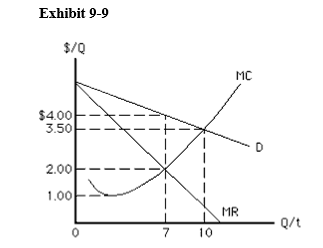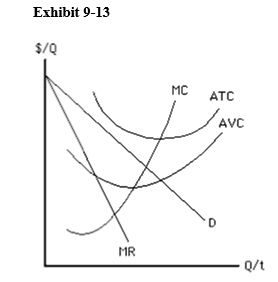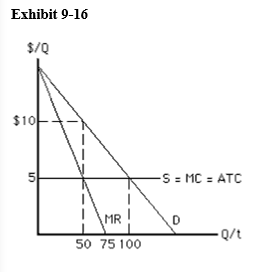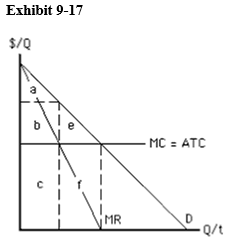##### Chapter 9 Practice MC

0
Set Details Share
created 4 years ago by melkojt
283 views
updated 4 years ago by melkojt
Subjects:
econ
Page to share:
Embed this setcancel
COPY
code changes based on your size selection
Size:
X
1

A monopolist is

1. one of a large number of small firms that produce a homogeneous good
2. one of a small number of large firms that produce a differentiated good
3. a single seller of a product with many close substitutes
4. one of a small number of large firms that produce a homogeneous good
5. a single seller of a product with no close substitutes

2

Patent laws

1. reduce incentive to innovate by restricting market entry
2. reduce incentive to innovate by making it difficult to use the patented innovation
3. increase incentive to innovate by restricting entry into a market
4. increase incentive to innovate by giving a firm permanent and exclusive production rights
5. give a firm the right to provide a wide variety of goods or services

3

Which of the following describes the market structure of monopoly?

1. many firms with some control over price, and considerable product differentiation
2. many firms with no control over price, producing identical products with no differentiation
3. a few firms with some control over price, producing similar products which are close substitutes
4. a few firms with no control over price, producing highly differentiated products
5. a single firm producing all of the output for the industry

4

A natural monopoly results when a firm has

2. a patent
3. official approval to produce a product
4. decreasing average costs over the range of market demand
5. exclusive use of a natural resource

5

If a firm is a natural monopoly, its

1. long-run average cost declines over the full range of market demand
2. long-run average cost increases over the full range of market demand
3. fixed cost declines over the full range of market demand
4. fixed cost increases over the full range of market demand
5. long-run average cost declines and marginal cost rises over the full range of market demand

6

The demand curve a monopolist uses in making an output decision is

1. the same as the demand curve facing a perfectly competitive firm
2. vertical because there are no close substitutes for its product
3. horizontal because there are no close substitutes for its product
4. the same as the market demand curve
5. perfectly inelastic

7

A monopolist's demand curve is

1. its marginal cost curve
2. its marginal revenue curve
3. identical to the market demand curve
4. the same as the demand curve of a firm in perfect competition
5. nonexistent

8

Which of the following is true of marginal revenue for a monopolist that charges a single price?

1. P = MR because there are no close substitutes for the monopolist's product.
2. P > MR because the monopolist must decrease price on all units sold in order to sell an additional unit.
3. P < MR because the monopolist must decrease price on all units sold in order to sell an additional unit.
4. AR = MR because there are no close substitutes for the monopolist's product.
5. P = MR only at the profit-maximizing quantity.

9

If a monopolist must lower the price on all units in order to sell an additional unit,

1. it is impossible for the monopolist to maximize profit
2. the monopolist will always lose profit when it increases quantity
3. the monopolist will always lose revenue when it increases quantity
4. price will always be greater than marginal revenue
5. price will always be less than marginal revenue

10

Suppose that a monopolist must choose between two points on its demand curve: it can sell 100 units for \$3 each, or it can sell 140 units for \$2 each. Which of the following is true?

1. The monopolist is facing elastic demand.
2. The monopolist is facing unit elastic demand.
3. The monopolist is facing inelastic demand.
4. The monopolist is facing perfectly elastic demand.
5. The elasticity of demand cannot be determined with the information given.

11

What is the relationship between price elasticity of demand and the monopolist's revenue?

1. marginal revenue is maximized where demand is unit elastic.
2. average revenue is maximized where demand is unit elastic.
3. marginal revenue is negative where demand is inelastic.
4. average revenue is negative where demand is inelastic.
5. marginal revenue is lowest where demand is unit elastic.

12

A profit-maximizing monopolist never produces along the __________ portion of the demand curve because marginal revenue is __________ there.

1. elastic; positive
2. elastic; negative
3. inelastic; negative
4. inelastic; positive
5. inelastic; zero

13

Negative marginal revenue means that

1. the firm is maximizing its economic profit
2. the firm is maximizing its total revenue
3. total revenue is increasing at an increasing rate as output increases
4. total revenue is increasing at a decreasing rate as output increases
5. total revenue is decreasing as output increases

14

Which of the following is not true of monopolists?

1. The entry of new firms is not a major concern.
2. Monopolists seek to maximize profits.
3. Monopolists can charge any price they want and make a profit.
4. Monopolists can choose any point on the market demand curve.
5. Monopolists can raise price more than 10 percent.

15

Which of the following is true at the profit-maximizing quantity for both a perfectly competitive firm and a monopoly?

1. Price equals marginal cost.
2. Price is greater than marginal cost.
3. Marginal revenue equals marginal cost.
4. Marginal revenue is less than marginal cost.
5. Marginal revenue is greater than average revenue.

16

A monopolist earning short-run economic profit determines that at its present level of output, marginal revenue is \$23 and marginal cost is \$30. Which of the following should the firm do to increase profit?

1. Raise price and lower output.
2. Lower price and lower output.
3. Raise price and raise output.
4. Lower price and raise output.
5. Lower output but leave price unchanged.

17

For a monopolist that does not price discriminate, economic profit is maximized in the short run at a price of \$140. Marginal revenue at that output level is

1. equal to \$140
2. greater than \$140
3. less than \$140
4. less than marginal cost
5. greater than average revenue

18

One likely result of monopoly power is

1. a wide variety of substitute products from which consumers can choose
2. an elimination of barriers to industry entry
3. a decline in government regulation
4. a higher price than would exist in a competitive industry
5. an improvement in allocative efficiency

19Assuming the firm should not shut down, the profit-maximizing price and quantity for the monopolist in Exhibit 9-9, which does not price discriminate, are

1. P = \$4 and Q = 7
2. P = \$3.50 and Q = 10
3. P = \$2 and Q = 7
4. P = \$1 and Q = 7
5. P = \$1 and Q = 10

20

A profit-maximizing monopolist that produces in the short run will

1. produce the level of output where marginal revenue exceeds marginal cost by the largest amount
2. increase output as long as the marginal revenue exceeds the marginal cost of producing that unit
3. produce the level of output where average total cost is at a minimum
4. increase price as long as the average revenue exceeds the average total cost
5. produce the level of output where average revenue exceeds average total cost by the largest amount

21

In the short run, a monopolist will shut down when

1. average total cost is greater than price at all output levels
2. average variable cost is greater than average fixed cost at all output levels
3. price is greater than average variable cost at all output levels
4. average fixed cost is greater than price at all output levels
5. average variable cost is greater than price at all output levels

22In the short run, the monopolist depicted in Exhibit 9-13 should

1. shut down because P < AVC at some output levels
2. shut down because P < ATC at all output levels
3. continue producing because P > AVC at some output levels
4. continue producing because P > ATC at all output levels
5. continue producing because monopolists never shut down

23

Barriers to entry

1. prevent monopolies from earning profit in the long run
2. prevent monopolies from earning profit in the short run
3. may allow monopolies to earn profit in the long run
4. prevent government from regulating a monopoly
5. prevent a natural monopoly from raising its price

24

The main reason a monopolist can earn long-run economic profit, whereas a perfectly competitive firm cannot, is that

1. monopolists operate under economies of scale
2. perfectly competitive firms have opportunity costs
3. demand for the monopolist's output is inelastic
4. demand for the monopolist's output is elastic
5. there are no barriers to entry in perfect competition

25

Firms can earn economic profits even in the long run if

1. they charge the highest price possible
2. there is a cost-reducing technological change
3. there are significant barriers to entry
4. marginal revenue equals marginal cost
5. price is less than average variable cost at all rates of output

26

An important difference between a perfectly competitive firm and a monopolist is

1. the perfectly competitive firm tends to be larger
2. only the monopolist attempts to maximize profit
3. only the perfectly competitive firm maximizes profit
4. the perfectly competitive firm faces a horizontal demand curve and the monopolist faces a downward-sloping demand curve
5. only the monopolist maximizes profit at the quantity where marginal cost equals marginal revenue

27

When compared to firms in perfect competition, monopolists tend to charge __________ prices and offer __________ quantities of output.

1. lower; lower
2. higher; lower
3. lower; higher
4. higher; higher
5. higher; the same

28Exhibit 9-16 depicts the cost and demand conditions facing a profit-maximizing monopolist that does not price discriminate. Which of the following statements is true?

1. An output of 50 is allocatively efficient, but the monopolist will produce 100 units.
2. An output of 50 is allocatively efficient, but the monopolist will produce 75 units.
3. An output of 75 is allocatively efficient, but the monopolist will produce 100 units.
4. An output of 100 is allocatively efficient, but the monopolist will produce 50 units.
5. An output of 100 is allocatively efficient, but the monopolist will produce 75 units.

29Exhibit 9-16 depicts the cost and demand conditions facing a profit-maximizing monopolist that does not price discriminate. What is the deadweight loss in this situation?

1. \$5
2. \$250
3. \$125
4. \$500
5. \$10

30What area in Exhibit 9-17 represents consumer surplus under monopoly without price discrimination?

1. area a
2. area b
3. area c
4. area a + b + e
5. area e

31Which area in Exhibit 9-17 represents monopoly profit without price discrimination?

1. area a
2. area b
3. area c
4. area b + e
5. area b + e + c + f

32Which area in Exhibit 9-17 represents deadweight loss under monopoly without price discrimination?

1. area a
2. area b
3. area c
4. area f
5. area e

33

A profit-maximizing monopolist produces an output level that is allocatively inefficient because

1. price is greater than marginal cost
2. price is less than marginal cost
3. marginal revenue is greater than marginal cost
4. marginal revenue is less than marginal cost
5. consumers wish to purchase all that is produced

34

If a perfectly competitive industry is monopolized, consumer surplus

1. can be expected to decrease
2. will usually remain constant
3. can be expected to increase
4. drops from a high value to zero
5. increases from zero to a high value

35

The welfare loss of monopoly is also called

1. converted consumer surplus
3. economic profit under monopoly
4. producer surplus
5. contestable profit

36

The practice of charging different prices to different consumers of the same product is called

1. monopolistic pricing
2. unit pricing
3. price discrimination
4. elasticity pricing
5. marginal cost pricing

37

A major fruit juice manufacturer failed in its attempt to engage in price discrimination between students and all other consumers. What is a possible explanation for this failure?

1. There was nothing to prevent the students from reselling the fruit juice to other consumers.
2. The fruit juice manufacturer produced in a perfectly competitive market.
3. The two groups of consumers probably have the same demand elasticity for fruit juice.
4. The cost of producing the product is relatively high.
5. Demand for fruit juice is probably inelastic.

38

For which of the following products would price discrimination be easiest?

1. orange juice
2. diamonds
3. compact disks
4. haircuts
5. gasoline

39

Suppose that a price-discriminating monopolist divides its market into two segments. If the firm sells its product for a price of \$42 in the market segment where demand is relatively less elastic, the price in the market segment whose customers' demand is more elastic will be

1. \$42
2. greater than \$42
3. less than \$42
4. less than marginal revenue in that market segment
5. equal to marginal revenue in that market segment

40

Suppose that a price-discriminating monopolist divides its market into two segments. The firm will charge the lower price in the market segment where consumers

1. have relatively less elastic demand
2. have relatively more elastic demand
3. attach a higher marginal value to each unit of the good
4. have perfectly inelastic demand
5. attach higher average value to units of the good

41

A monopolist that engages in perfect price discrimination

1. divides all buyers into two mutually exclusive groups
2. refuses to sell to consumers of certain races, sexes, or creeds
3. charges the same price for every unit sold
4. charges a different price for every unit sold
5. charges buyers who want a little of the good a low price and charges buyers who want a lot of the good a high price